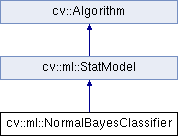OpenCV  3.2.0 Open Source Computer Vision
cv::ml::NormalBayesClassifier Class Referenceabstract

Bayes classifier for normally distributed data. More...

#include "ml.hpp"

Inheritance diagram for cv::ml::NormalBayesClassifier:## Public Member Functions

virtual float predictProb (InputArray inputs, OutputArray outputs, OutputArray outputProbs, int flags=0) const =0
Predicts the response for sample(s). More...Public Member Functions inherited from cv::ml::StatModel
virtual float calcError (const Ptr< TrainData > &data, bool test, OutputArray resp) const
Computes error on the training or test dataset. More...

virtual bool empty () const
Returns true if the Algorithm is empty (e.g. in the very beginning or after unsuccessful read. More...

virtual int getVarCount () const =0
Returns the number of variables in training samples. More...

virtual bool isClassifier () const =0
Returns true if the model is classifier. More...

virtual bool isTrained () const =0
Returns true if the model is trained. More...

virtual float predict (InputArray samples, OutputArray results=noArray(), int flags=0) const =0
Predicts response(s) for the provided sample(s) More...

virtual bool train (const Ptr< TrainData > &trainData, int flags=0)
Trains the statistical model. More...

virtual bool train (InputArray samples, int layout, InputArray responses)
Trains the statistical model. More...Public Member Functions inherited from cv::Algorithm
Algorithm ()

virtual ~Algorithm ()

virtual void clear ()
Clears the algorithm state. More...

virtual String getDefaultName () const

virtual void read (const FileNode &fn)
Reads algorithm parameters from a file storage. More...

virtual void save (const String &filename) const

virtual void write (FileStorage &fs) const
Stores algorithm parameters in a file storage. More...

## Static Public Member Functions

static Ptr< NormalBayesClassifiercreate ()Static Public Member Functions inherited from cv::ml::StatModel
template<typename _Tp >
static Ptr< _Tp > train (const Ptr< TrainData > &data, int flags=0)
Create and train model with default parameters. More...Static Public Member Functions inherited from cv::Algorithm
template<typename _Tp >
static Ptr< _Tp > load (const String &filename, const String &objname=String())
Loads algorithm from the file. More...

template<typename _Tp >
static Ptr< _Tp > loadFromString (const String &strModel, const String &objname=String())
Loads algorithm from a String. More...

template<typename _Tp >
static Ptr< _Tp > read (const FileNode &fn)
Reads algorithm from the file node. More...Public Types inherited from cv::ml::StatModel
enum  Flags {
UPDATE_MODEL = 1,
RAW_OUTPUT =1,
COMPRESSED_INPUT =2,
PREPROCESSED_INPUT =4
}Protected Member Functions inherited from cv::Algorithm
void writeFormat (FileStorage &fs) const

## Detailed Description

Bayes classifier for normally distributed data.

Normal Bayes Classifier

## § create()

 static Ptr cv::ml::NormalBayesClassifier::create ( )
static

Creates empty model Use StatModel::train to train the model after creation.

## § predictProb()

 virtual float cv::ml::NormalBayesClassifier::predictProb ( InputArray inputs, OutputArray outputs, OutputArray outputProbs, int flags = 0 ) const
pure virtual

Predicts the response for sample(s).

The method estimates the most probable classes for input vectors. Input vectors (one or more) are stored as rows of the matrix inputs. In case of multiple input vectors, there should be one output vector outputs. The predicted class for a single input vector is returned by the method. The vector outputProbs contains the output probabilities corresponding to each element of result.

The documentation for this class was generated from the following file: